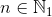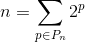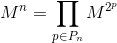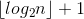# The Nth Fibonacci Number in O(log N)

Algorithms

Find the Nth Fibonacci Number in O(N) time of arithmetic operations.

Thinking about it, I realized that the only solutions coming to my mind were those operating in O(n) time. But I found a better solution later.

I am going to use the following denotation of sets:- non-negative integers— positive integers

According to various mathematic traditions, a set of natural numbers can either contain 0 or not. Therefore, it’s preferred to indicate it explicitly in international mathematic texts.

## The Solution

Donald E. Knuth provides a matrix identity of the following form:The identity is provided without any proof, but it’s quite easy to prove it by induction.

The matrix on the right is called Q-matrix.

Let’s indicate:According to the identity,. Thus, to calculate, we should calculatematrix and take the first element of the first line (the enumeration starts with 1).

Sincecalculation comes to raising the matrix to power, let’s take a look at this process in details.

Let’s say there is amatrix to be raised topower. Suppose,is the power of 2. Thus,.

We can representin the form of a tree:Meaning that:So, to calculatematrix, we should calculatematrix and multiply it by itself. To calculatewe would have to do the same withand so on.

Obviously, the tree height is.

Let’s estimatecalculation time.matrix is of the same size in any power. Therefore, we can perform the multiplication of two matrices in any power in. We should performof such multiplications. So,complexity is.

### But what if n is not a power of 2?

There is a question now: what should we do ifis not a power of 2? We can factor down any natural numberas a sum of numbers that are the power of 2, without any repetitions (we do it all the time when converting a number from a binary to decimal numeral system). I.e.:whereis a set of powers, with the help of which we express. Remembering thatis the power of a matrix, we get:.

Matrix multiplication is not commutative though, i.e. the order of operands during the multiplication is important. But the commutative property is kept for the so-called permutation matrices. Matrixis permutational for,. Thus, we will not have to consider the order of operands during the multiplication task, which simplifies the process a bit.

So, we can represent the algorithm ofcalculation if the form of the following two steps:

1. Factor downinto the sum of the powers of two in the form of.
2. Calculate all the elements ofset.
3. Calculate.

Let’s estimate the execution time of the given algorithm.

The first step performs in, whereis the number of binary digits in.

Since we have to perform no more thanmatrix exponentiation, the second step takestime.

The third step performs in, as we should multiply matrices no more thantimes.

## Optimization

Is it possible to improve the algorithm execution time? Yes, it is. You should note that the second step does not describe the method of calculating matrices that belong toset. We know thatis the power of 2 for each of them. Getting back to the picture with the tree, it means that all of them lie in some or other tree tiers and to calculate the greater ones, we should calculate the inferior ones. If we apply Memoization technique and cache all the calculated matrices of every tier, this will allow us to shorten the execution time of the second step up to, as well as the total execution time of a whole algorithm. That’s exactly what we need according to the task.

## The Code

Let’s get down to coding. I implemented the algorithm in Python due to the following reasons:

It’s going to look like this:

``````class MatrixFibonacci:
Q = [[1, 1],
[1, 0]]

def __init__(self):
self.__memo = {}

def __multiply_matrices(self, M1, M2):
"""Matrices miltiplication
(the matrices are expected in the form of a list of 2x2 size)."""

a11 = M1*M2 + M1*M2
a12 = M1*M2 + M1*M2
a21 = M1*M2 + M1*M2
a22 = M1*M2 + M1*M2
r = [[a11, a12], [a21, a22]]
return r

def __get_matrix_power(self, M, p):
"""Matrix exponentiation (it is expected that p that is equal to the power of 2)."""

if p == 1:
return M
if p in self.__memo:
return self.__memo[p]
K = self.__get_matrix_power(M, int(p/2))
R = self.__multiply_matrices(K, K)
self.__memo[p] = R
return R

def get_number(self, n):
"""Getting the nth Fibonacci number
(a non-negative integer number is expected as n)."""
if n == 0:
return 0
if n == 1:
return 1
# Factoring down the passed power into the powers that are equal to the power of 2),
# i.e. 62 = 2^5 + 2^4 + 2^3 + 2^2 + 2^0 = 32 + 16 + 8 + 4 + 1.
powers = [int(pow(2, b))
for (b, d) in enumerate(reversed(bin(n-1)[2:])) if d == '1']
# The same, but less pythonic: http://pastebin.com/h8cKDkHX

matrices = [self.__get_matrix_power(MatrixFibonacci.Q, p)
for p in powers]
while len(matrices) > 1:
M1 = matrices.pop()
M2 = matrices.pop()
R = self.__multiply_matrices(M1, M2)
matrices.append®
return matrices

mfib = MatrixFibonacci()
for n in range(0, 128):
num = mfib.get_number(n)
print(num)
``````

## Benchmarks

I wanted to compare the execution time of my algorithm with a classical iteration algorithm that is based on the consecutivecalculation saving the previous two numbers. I implemented it like this:

``````def get_number(self, n):
if n == 0:
return 0
a = 0
b = 1
c = 1
for i in range(n-1):
c = a + b
a = b
b = c
return c

``````

I used the following technique of performance testing.number acceptsvalues. Objects of MatrixFibonacci and IterationFibonacci (it’s the class that calculates Fibonacci numbers iteratively) classes are created for each. Then, an arbitraryinteger is generated 10 000 times.

The test outputs a lot of strings like: n T1 T2. These data is used for building a chart.As you can see from the chart, the matrix algorithm leaves the iterative algorithm behind starting from(its worth noting thatwill not fit into unsigned 64 bits). I guess, it’s possible to say that the algorithm is impractical, though its theoretical speed is quite good.

You will find the complete source code with the benchmark here.

The code for gnuplot to build charts:

``````set terminal png
set output 'fibgr.png'
set grid
set style data linespoints
set title "Calculation of 10000 Fibonacci numbers (Fn)"
set xlabel "Approximate number n"
set ylabel "Time, s"
plot "benchmark.txt" using 1:2 with lines title 'iterative', \
"benchmark.txt" using 1:3 with lines title 'matrix'
``````
• Donald E. Knuth. The Art of Computer Programming. Volume 1. Fundamental Algorithms.
3,751

## Ropes — Fast Strings

Most of us work with strings one way or another. There’s no way to avoid them — when writing code, you’re doomed to concatinate strings every day, split them into parts and access certain characters by index. We are used to the fact that strings are fixed-length arrays of characters, which leads to certain limitations when working with them. For instance, we cannot quickly concatenate two strings. To do this, we will at first need to allocate the required amount of memory, and then copy there the data from the concatenated strings.